Ask question

# Proving Similarity in the figure DEFG is a square. Prove the following: /_ADG~/_GCF Given: The given figure is, 12210202691.jpg# Proving Similarity in the figure DEFG is a square. Prove the following: /_ADG~/_GCF Given: The given figure is, 12210202691.jpg

Question
Similarityasked 2021-02-02
Proving Similarity in the figure DEFG is a square. Prove the following:
$$\displaystyle\triangle{A}{D}{G}\sim\triangle{G}{C}{F}$$
Given:
The given figure is,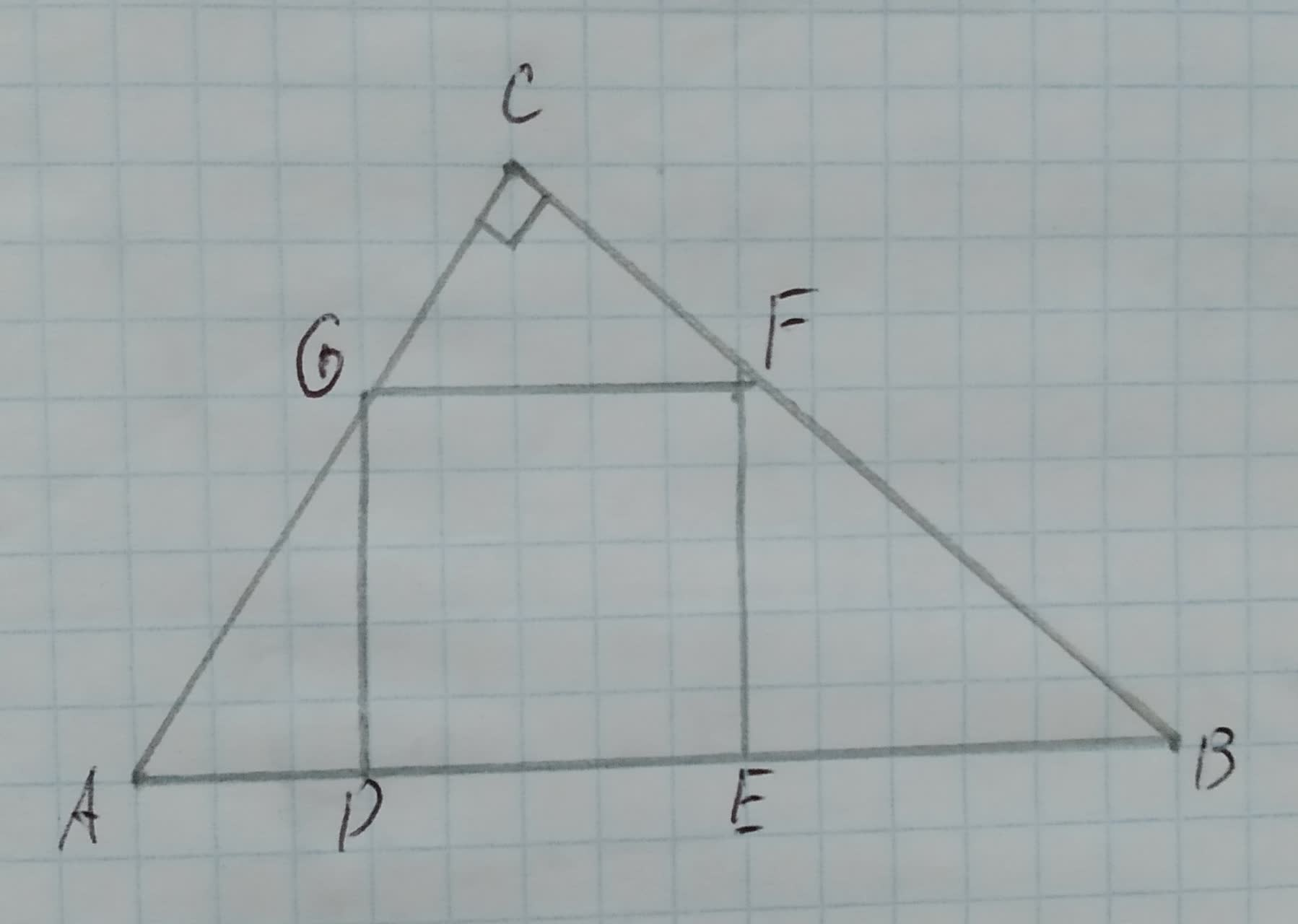## Answers (1)2021-02-03
Approach:
Two triangles are similar if their vertices can be matched up so that corresponding angles are congruent. In this case corresponding sides are proportional.
If the two angles of the triangles are same then the third angle of the triangles have to be same,because the sum of angles in a triangle is $$\displaystyle{180}^{{\circ}}$$. Therefore, the triangles are similar by AA rule if two angles are same.
Calculation:
It is given that DEFG is a square.
Consider $$\displaystyle\triangle{A}{D}{G}{\quad\text{and}\quad}\triangle{G}{C}{F}$$.
$$\displaystyle\angle{A}{D}{G}=\angle{G}{C}{F}$$ both are $$\displaystyle{90}^{{\circ}}$$.
And $$\displaystyle{G}{F}{\mid}{\mid}{A}{B}$$, thus, $$\displaystyle\angle{C}{G}{F}=\angle{G}{A}{D}$$ as they are alternate angles.
Therefore, $$\displaystyle\triangle{A}{D}{G}\sim\triangle{G}{C}{F}$$ by AA rule.
Conclusion:
Hence, it is proved that $$\displaystyle\triangle{A}{D}{G}\sim\triangle{G}{C}{F}$$.

### Relevant Questionsasked 2020-11-08
Proving Similarity in the figure DEFG is a square. Prove the following:
$$\displaystyle\triangle{A}{D}{G}\sim\triangle{F}{E}{B}$$
Given:
The given figure is,asked 2020-10-23
Proving Similarity in the figure DEFG is a square. Prove the following:
$$\displaystyle{D}{E}=\sqrt{{{A}{D}\cdot{E}{B}}}$$
Given:
The given figure is,asked 2020-11-29
Proving Similarity in the figure DEFG is a square. Prove the following:
AD*EB=DG*FE
Given:
The given figure is,asked 2021-02-21
To prove: The similarity of $$\displaystyle\triangle{N}{W}{O}$$ with respect to $$\displaystyle\triangle{S}{W}{T}$$.
Given information: Here, we have given that NPRV is a parallelogram.asked 2021-05-16
To prove : The similarity of $$\displaystyle\triangle{N}{R}{T}$$ with respect to $$\displaystyle\triangle{N}{S}{P}$$.
Given information: Here, we have given that $$\displaystyle\overline{{{S}{P}}}$$ is altitude to $$\displaystyle\overline{{{N}{R}}}\ {\quad\text{and}\quad}\ \overline{{{R}{T}}}$$ is altitude to $$\displaystyle\overline{{{N}{S}}}$$.asked 2021-04-16
To prove: The similarity of $$\displaystyle\triangle{B}{C}{D}$$ with respect to $$\displaystyle\triangle{F}{E}{D}$$.
Given information: Here, we have given that $$\displaystyle\overline{{{A}{C}}}\stackrel{\sim}{=}\overline{{{A}{E}}}\ {\quad\text{and}\quad}\ \angle{C}{B}{D}\stackrel{\sim}{=}\angle{E}{F}{D}$$asked 2020-11-01
Proving Similarity In the figure CDEF is a rectangle. Prove that $$\displaystyle\triangle{A}{B}{C}\sim\triangle{E}{B}{F}$$.
Given:
The given figure is,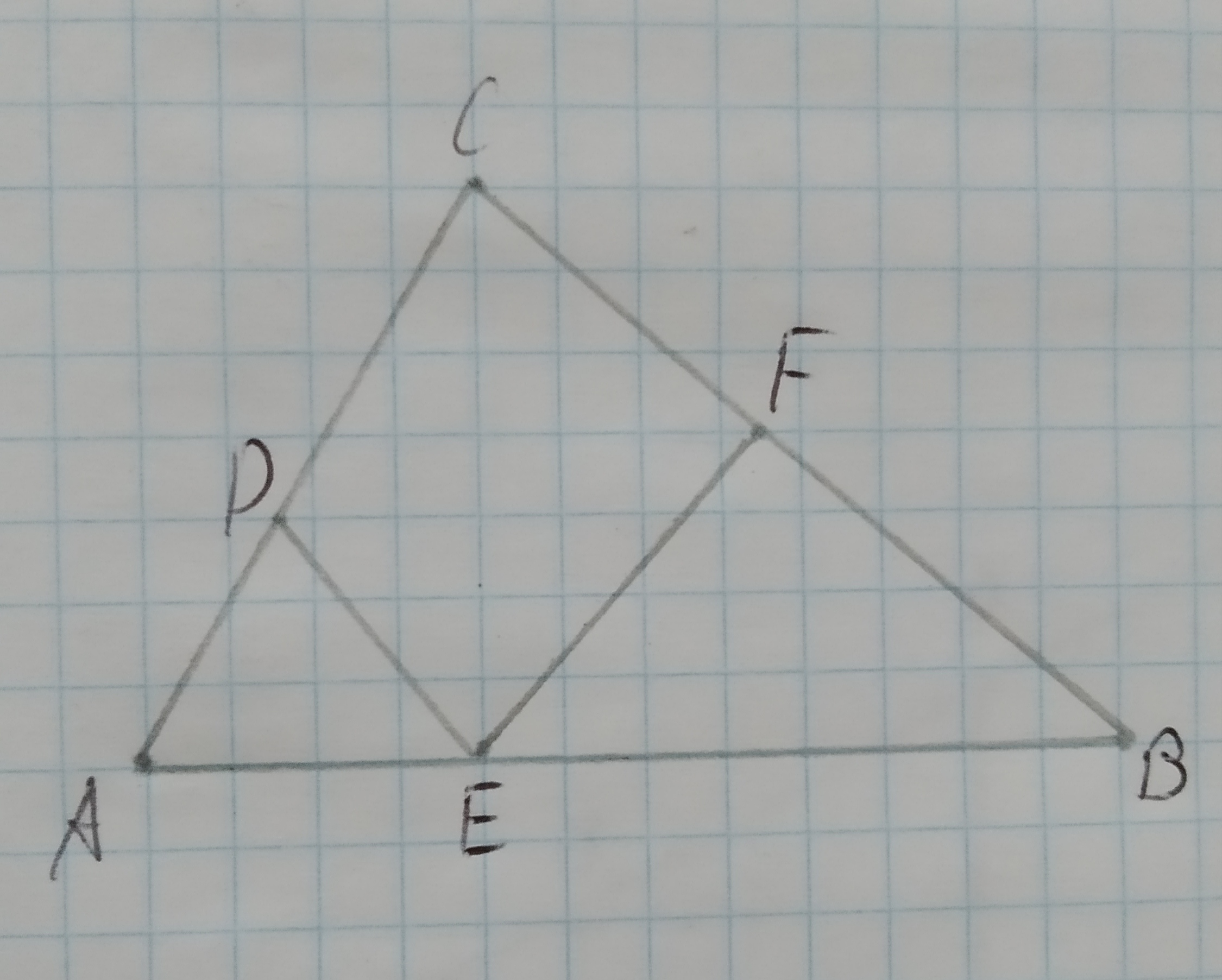asked 2021-01-28
To check: whether the additional information in the given option would be enough to prove the given similarity.
Given:
The given similarity is $$\displaystyle\triangle{A}{D}{C}\sim\triangle{B}{E}{C}$$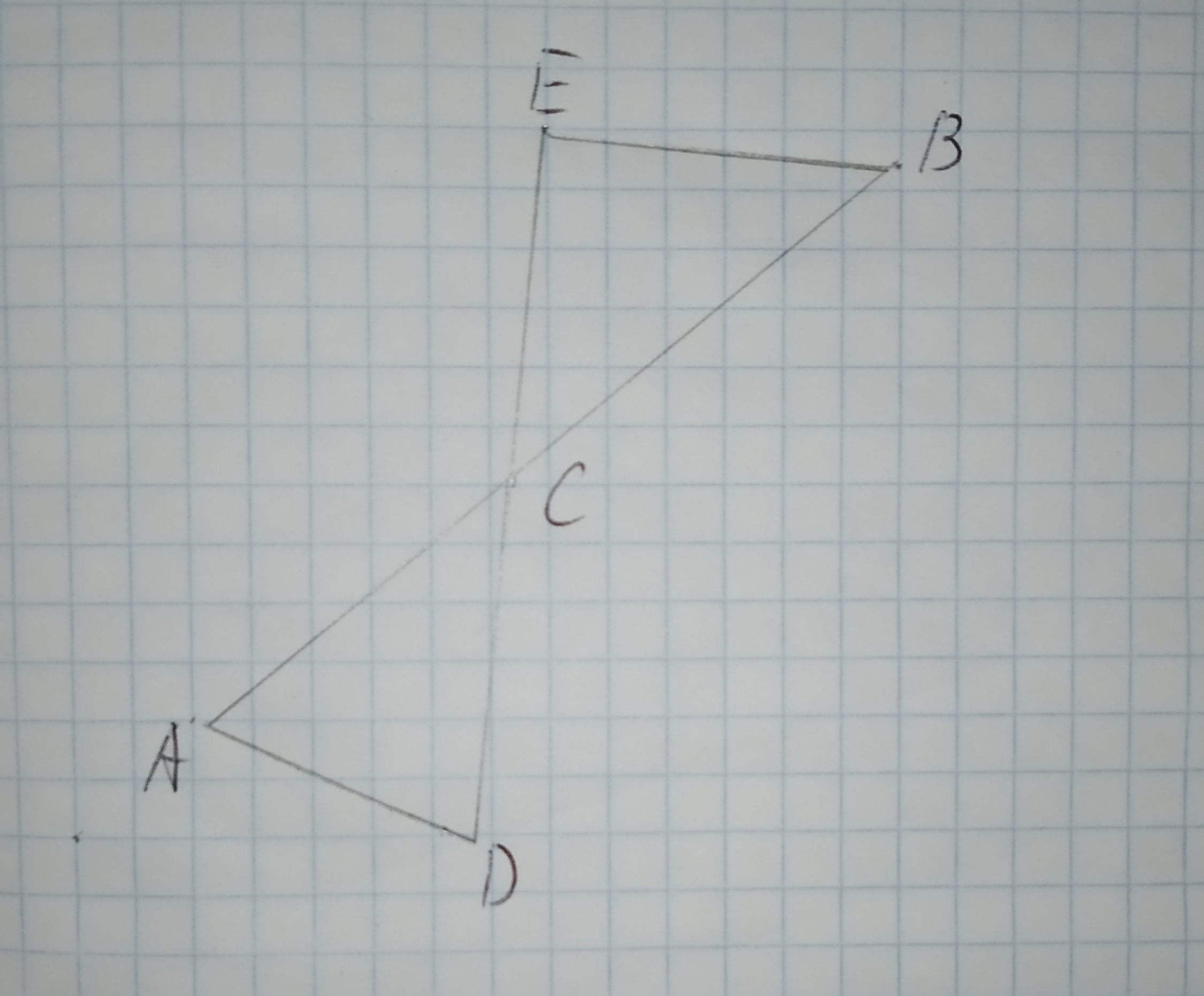The given options are:
A.$$\displaystyle\angle{D}{A}{C}{\quad\text{and}\quad}\angle{E}{C}{B}$$ are congruent.
B.$$\displaystyle\overline{{{A}{C}}}{\quad\text{and}\quad}\overline{{{B}{C}}}$$ are congruent.
C.$$\displaystyle\overline{{{A}{D}}}{\quad\text{and}\quad}\overline{{{E}{B}}}$$ are parallel.
D.$$\displaystyle\angle{C}{E}{B}$$ is a right triangle.asked 2020-12-06
To determine:Whether each pair of figures is similar and also write similarity statement and scale factor.
Given information:
Given the figure.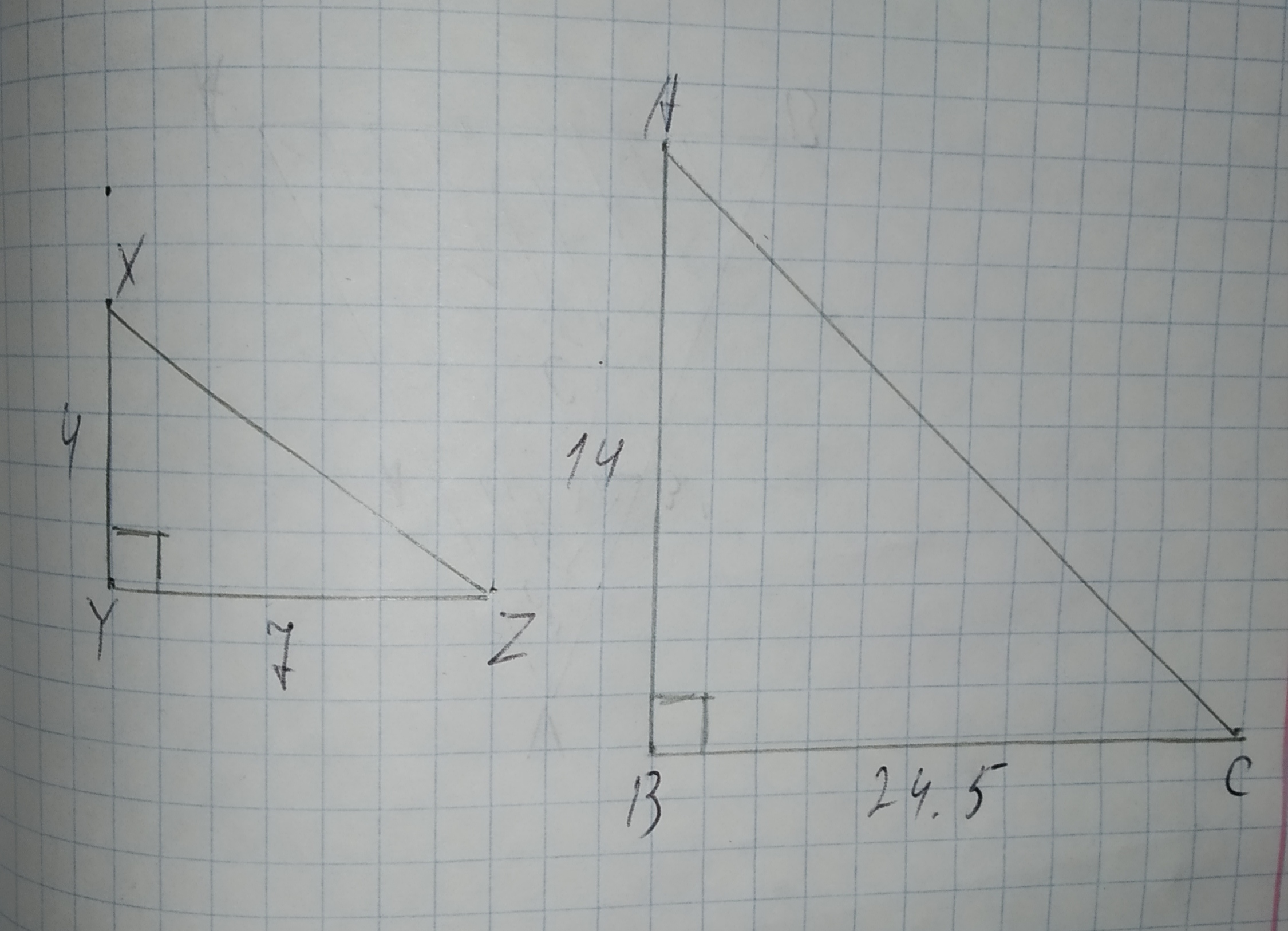asked 2020-12-12
Whether the triangle are similar. if so write the similarity statement and if not what would be the sufficient to prove the triangle similar.
Given: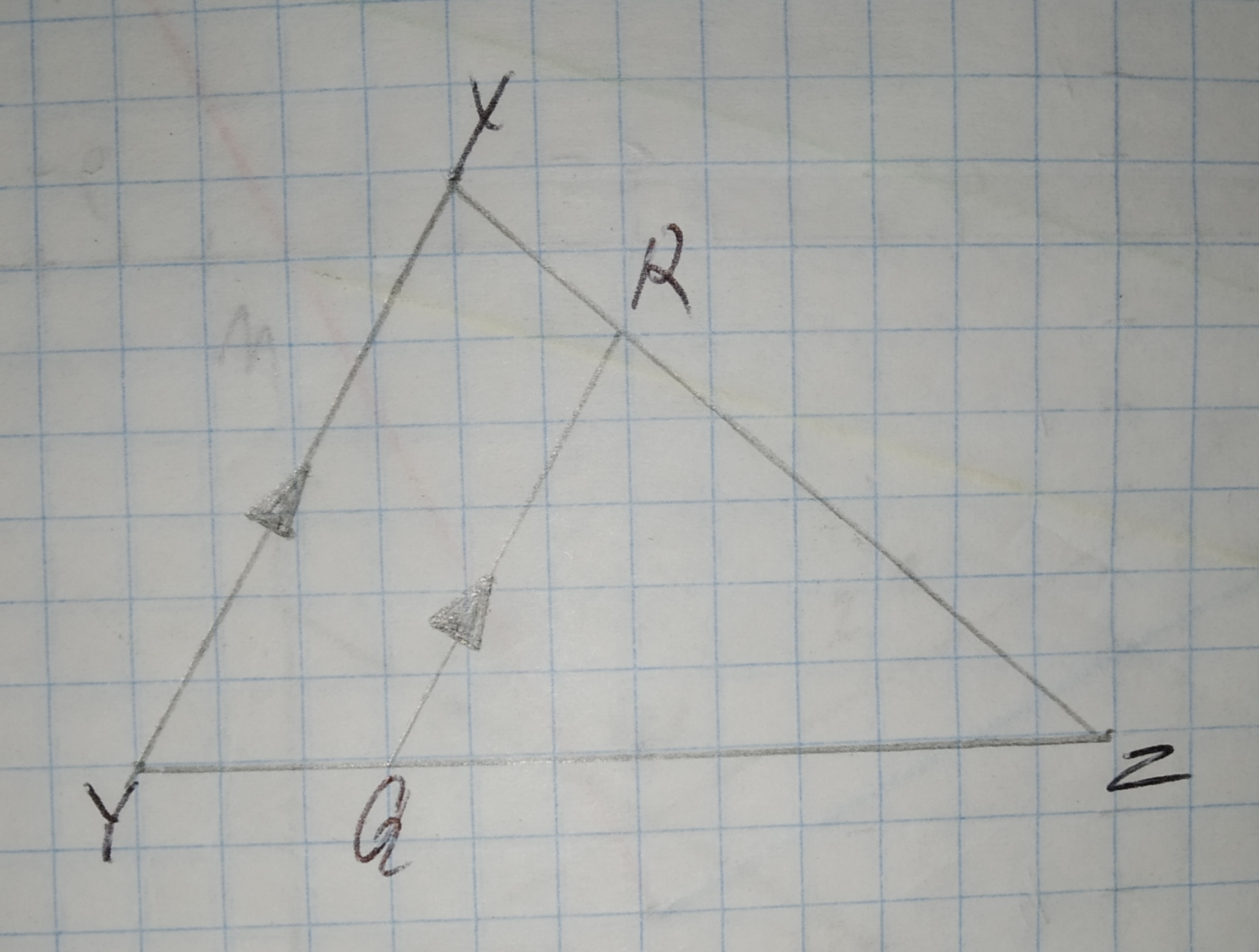...